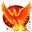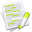#wx.lib.plot.polyobjects.PolyBoxPlot¶

Creates a PolyBoxPlot object.

param data

Raw data to create a box plot from.

type data

sequence of int or float

param **attr

keyword attributes

Keyword and Default

Description

Type

colour='black'

edge color

wx.Colour

width=1

edge width

float

style=wx.PENSTYLE_SOLID

edge style

wx.PenStyle

legend=''

legend string

str

Note

np.NaN and np.inf values are ignored.

TODO

• [ ] Figure out a better way to get multiple box plots side-by-side (current method is a hack).

• [ ] change the X axis to some labels.

• [ ] Change getClosestPoint to only grab box plot items and outlers? Currently grabs every data point.

• [ ] Add more customization such as Pens/Brushes, outlier shapes/size, and box width.

• [ ] Figure out how I want to handle log-y: log data then calcBP? Or should I calc the BP first then the plot it on a log scale?

##Class Hierarchy¶Inheritance diagram for class PolyBoxPlot:

##Known Superclasses¶

wx.lib.plot.polyobjects.PolyPoints

##Methods Summary¶

 __init__ Initialize self. See help(type(self)) for accurate signature. boundingBox Returns bounding box for the plot. calcBpData Box plot points calcOutliers Calculates the outliers. Must be called after calcBpData. draw Draws a box plot on the DC. getClosestPoint Returns the index of closest point on the curve, pointXY, getSymExtent Width and Height of Marker

##Class API¶

class PolyBoxPlot(PolyPoints)

Creates a PolyBoxPlot object.

Parameters
• data (sequence of int or float) – Raw data to create a box plot from.

• **attr – keyword attributes

Keyword and Default

Description

Type

colour='black'

edge color

wx.Colour

width=1

edge width

float

style=wx.PENSTYLE_SOLID

edge style

wx.PenStyle

legend=''

legend string

str

Note

np.NaN and np.inf values are ignored.

TODO

• [ ] Figure out a better way to get multiple box plots side-by-side (current method is a hack).

• [ ] change the X axis to some labels.

• [ ] Change getClosestPoint to only grab box plot items and outlers? Currently grabs every data point.

• [ ] Add more customization such as Pens/Brushes, outlier shapes/size, and box width.

• [ ] Figure out how I want to handle log-y: log data then calcBP? Or should I calc the BP first then the plot it on a log scale?

### Methods¶

__init__(self, points, **attr)

Initialize self. See help(type(self)) for accurate signature.

boundingBox(self)

Returns bounding box for the plot.

Override method.

calcBpData(self, data=None)

Box plot points:

Median (50%) 75% 25% low_whisker = lowest value that’s >= (25% - (IQR * 1.5)) high_whisker = highest value that’s <= 75% + (IQR * 1.5)

outliers are outside of 1.5 * IQR

dataarray-like

The data to plot

bpdatacollections.namedtuple

Descriptive statistics for data: (min_data, low_whisker, q25, median, q75, high_whisker, max_data)

calcOutliers(self, data=None)

Calculates the outliers. Must be called after calcBpData.

draw(self, dc, printerScale, coord=None)

Draws a box plot on the DC.

The following draw order is required:

1. First the whisker line

2. Then the IQR box

3. Lasly the median line.

This is because

• The whiskers are drawn as single line rather than two lines

• The median line must be visable over the box if the box has a fill.

Other than that, the draw order can be changed.

getClosestPoint(self, pntXY, pointScaled=True)

Returns the index of closest point on the curve, pointXY, scaledXY, distance x, y in user coords.

Override method.

if pointScaled == True, then based on screen coords if pointScaled == False, then based on user coords

getSymExtent(self, printerScale)

Width and Height of Marker